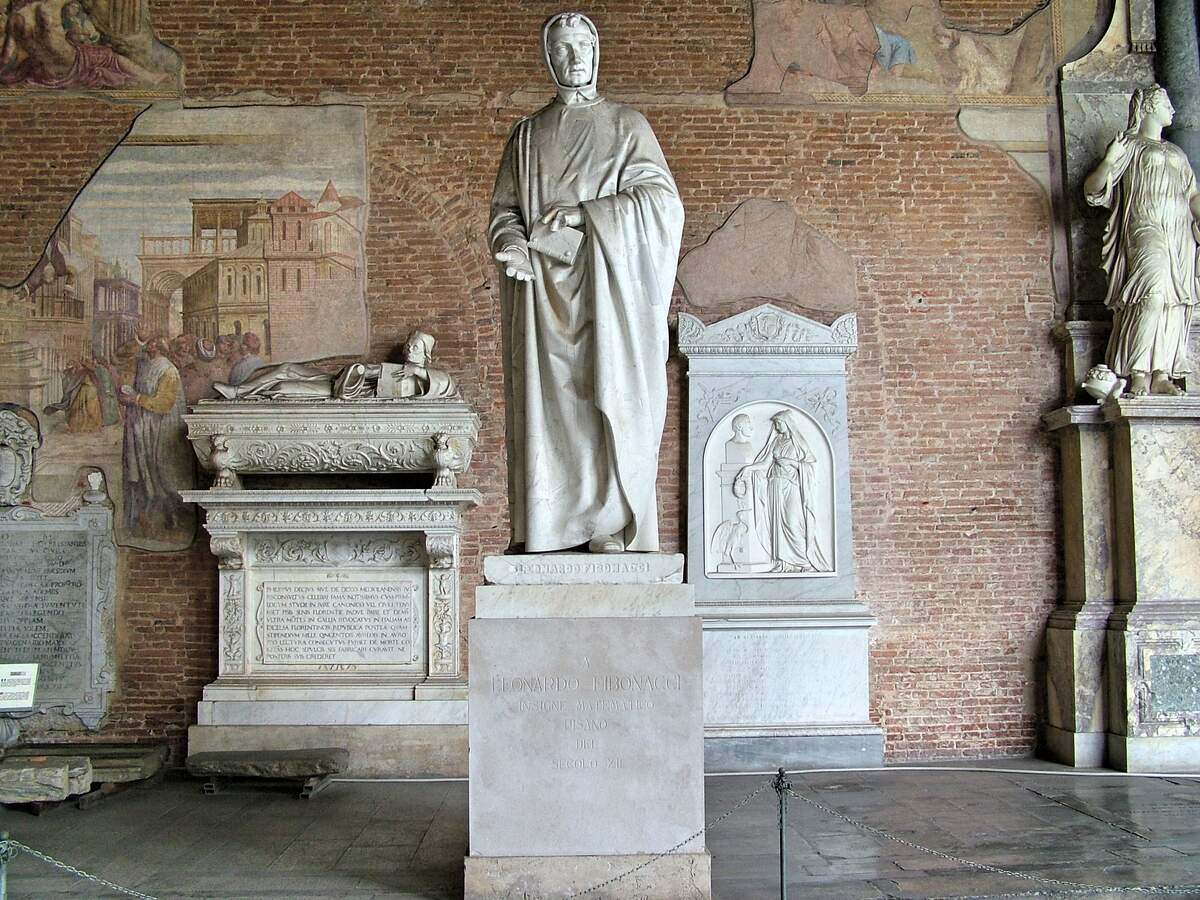# Fibonacci Day

## Sources

• ### https://www.livescience.com/37470-fibonacci-sequence.html

November 23—or 11/23—is the date of Fibonacci Day because the first series of numbers in the Fibonacci sequence are 1, 1, 2, and 3. The sequence is created by adding the previous two numbers to get the third number, so it begins as follows: 1, 1, 2, 3, 5, 8, 13, 21, 34, 55, 89, and 144. Commonly studied in high school and college classes, it can be described with the mathematical equation Xn+2 = Xn+1 + Xn.

The Fibonacci sequence can be visualized by viewing a logarithmic spiral called the Fibonacci spiral. The spiral is made by using tiling squares with consecutive Fibonacci numbers, where an arc is drawn connecting the opposite corners of each tile and then into the next tile. The numbers of the Fibonacci sequence approximate the golden ratio, an irrational number that goes to infinity, and the Fibonacci spiral approximates the golden spiral.

The Fibonacci sequence is named after Leonardo Pisano Bigollo, an Italian mathematician from the city of Pisa, born around 1170 CE, who went by many other names, such as Leonardo of Pisa, Leonardo Bonacci, and later, as Leonardo Fibonacci (which means "son of Bonacci" or "son of the Bonacci clan"), or simply, Fibonacci. His father was a businessman and merchant from Pisa, and he grew up in a trading colony in North Africa, where arithmetic was crucial to keep track of transactions. Young Fibonacci learned the Hindu-Arabic numeral system there, which was a more efficient system than Roman numerals, and today is the most used system in the world.

In 1202, Fibonacci published Liber Abaci. Written for tradesmen, it displayed the superiority of the Hindu-Arabic numeral system to the Roman numeral system and showed how the Hindu-Arabic system could be beneficial to Italian merchants for tasks like tracking profits, losses, and remaining loan balances. It was also in this book that Fibonacci laid out what would become known as the Fibonacci sequence. Although he didn't discover it—it can be found in ancient Sanskrit texts centuries before his time—he helped to popularize it, by being the first to introduce it to the Western world. He introduced it by asking a question related to rabbits: "A certain man put a pair of rabbits in a place surrounded on all sides by a wall. How many pairs of rabbits can be produced from that pair in a year if it is supposed that every month each pair begets a new pair which from the second month on becomes productive?" The formula that is used to find that answer is the Fibonacci sequence.

Beyond the small section in Liber Abaci, Fibonacci didn't mention the sequence, and it was rarely brought up by others until the nineteenth century. It was given the name "the Fibonacci sequence" by French mathematician Édouard Lucas in 1877. Today it is often spotted in nature, and has been referred to as "nature's numbering system" or "nature's universal rule." Numerous plants have Fibonacci spirals in their leaves and petals, and pinecones and sunflower seeds have them. The sequence is also found in the shapes of hurricanes and galaxies, and even in music. It is commonly postulated that the nautilus seashell has it, but it does not; there are other similar claims that go too far. Still, we celebrate the sequence and many places it can be found today, on Fibonacci Day!

## How to Observe Fibonacci Day

A few ideas on how to observe Fibonacci Day include:

## Exclusive Content

Enjoying Checkiday? It takes a lot of support from fans like you to run a free website. For exclusive content and other perks, please consider supporting us on Patreon. Thank you!

This event does not currently have a sponsor. If you'd like to increase visibility for this event while gaining exposure for yourself or your brand, you can learn more here!